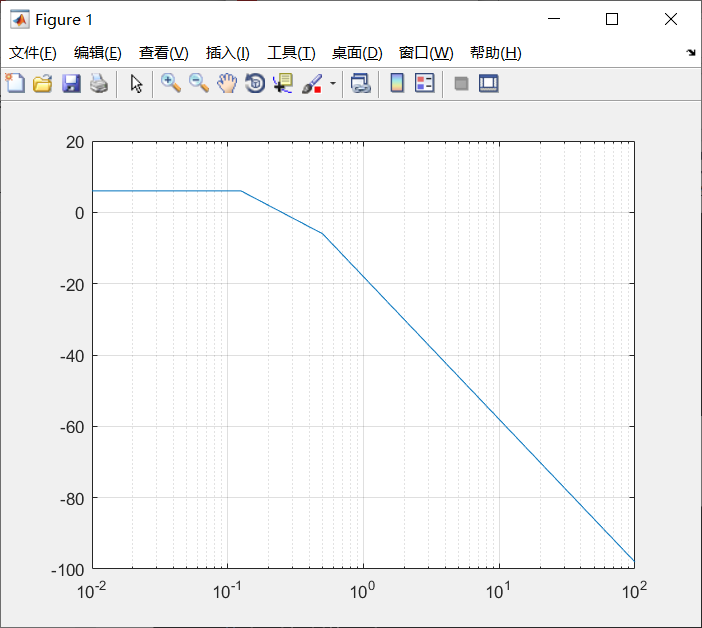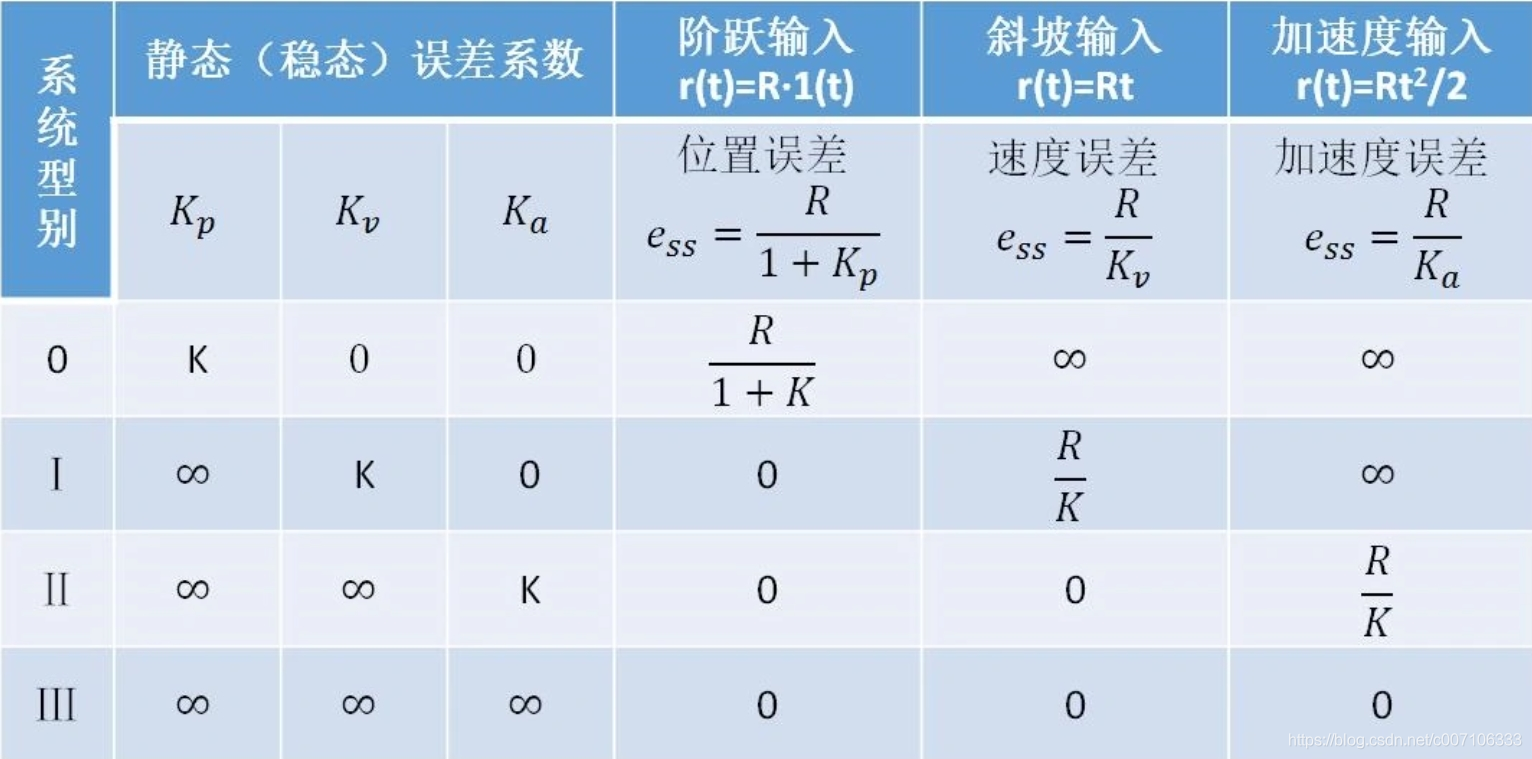• function[wpos,ypos]=bd_asymp(G,w) G1=zpk(G); wpos=[]; pos1=[]; if nargin==1,w=freqint2(G); end zer=G1.z{1}; pol=G1.p{1}; gain=G1.k; for i=1:length(zer); if isreal(zer(i)) ... if ima
function[wpos,ypos]=bd_asymp(G,w)
G1=zpk(G);
wpos=[];
pos1=[];
if nargin==1,w=freqint2(G);
end
zer=G1.z{1}; pol=G1.p{1};
gain=G1.k;
for i=1:length(zer);
if isreal(zer(i))
wpos=[wpos,abs(zer(i))];
pos1=[pos1,20];
else
if imag(zer(i))>0
wpos=[wpos,abs(zer(i))];
pos1=[pos1,40];
end
end
end
for i=1:length(pol);
if isreal(pol(i))
wpos=[wpos,abs(pol(i))];
pos1=[pos1,-20];
else
if imag(pol(i))>0
wpos=[wpos,abs(pol(i))];
pos1=[pos1,-40];
end
end
end
wpos=[wpos w(1) w(length(w))];
pos1=[pos1,0,0];
[wpos,ii]=sort(wpos);
pos1=pos1(ii);
ii=find(abs(wpos)<eps);
kslp=0;
w_start=1000*eps;
if length(ii)>0
kslp=sum(pos1(ii));
ii=(ii(length(ii))+1):length(wpos);
wpos=wpos(ii);
pos1=pos1(ii);
end
while 1
[ypos1,pp]=bode(G,w_start);
if isinf(ypos1),w_start=w_start*10;
else break;
end
end
wpos=[w_start wpos];
ypos(1)=20*log10(ypos1);
pos1=[kslp pos1];
for i=2:length(wpos)
kslp=sum(pos1(1:i-1));
ypos(i)=ypos(i-1)+kslp*log10(wpos(i)/wpos(i-1));
end
ii=find(wpos>=w(1)&wpos<=w(length(w)));
wpos=wpos(ii);
ypos=ypos(ii);
调用:
G1=tf(2,[conv([2,1],[8,1])]);
w=10e-3:0.1:100;
[x1,y1]=bd_asymp(G1,w);
semilogx(x1,y1),grid;展开全文matlab
• 绘制传递函数为G(s)=75*(0.2s+1)/(s(s^2+16s+100))的对数幅频特性。 重点： 提取分子分母的各项系数，将分子分母都展开，分子：15s+75，分母：s^3+16*s ^2 +100s. 分子各项系数分别为：0，0,15,75 分母各项系数分别...
题目：
绘制传递函数为G(s)=75*(0.2s+1)/(s(s^2+16s+100))的对数幅频特性。
重点：
提取分子分母的各项系数，将分子分母都展开，分子：15s+75，分母：s^3+16*s ^2 +100s. 分子各项系数分别为：0，0,15,75 分母各项系数分别为：1，16,100,0
代码：
num=[0 0 15 75];
den=[1 16 100 0];
w=logspace(-2,3,1000);
bode(num,den,w);
title('G(s)=75*(0.2*s+1)/(s*(s^2+16s+100))');
grid on;

结果展示：展开全文程序人生
• 不同阻尼比情况下幅频曲线（放大因子随频率比变化曲线）和相频曲线结构动力学
• 有源滤波器幅频特性曲线对数坐标纸，可以直接打印出来绘制有源滤波器特性曲线
• 五，高阶系统 从以上分析来看，二阶系统通过幅频特性曲线来分析系统动态性能，没有时域分析来的简单直观，但高阶是系统即使用时域法降阶分析也挺麻烦，当然三阶以上的系统要求出频域的γ和ωc也不容易。因此我们的...
前边的文章介绍过在时域上系统的特征根对系统特性的影响： 二阶系统欠阻尼状态极点位置对阶跃响应的影响 这里简单回顾下： 典型二阶系统的传递函数如下：

H

(

s

)

=

ω

n

2

s

2

+

2

ξ

∗

ω

n

∗

s

+

ω

n

2

\ H(s)= \frac{ωn^2 }{s^2 +2ξ*ωn*s+ωn^2}

特征方程为：

s

2

+

2

ξ

∗

ω

n

∗

s

+

ω

n

2

=

0

\ {s^2 +2ξ*ωn*s+ωn^2}=0

欠阻尼状态下的特征根为：

s

1

,

2

=

−

ξ

∗

ω

n

±

j

w

n

∗

1

−

ξ

2

(

0

<

ξ

<

1

)

\mathop{{s}} \nolimits_{{1,2}} = {-ξ*ωn \pm j wn*\sqrt{1-ξ^2}}(0<ξ<1)

特征根的实部决定了系统的稳定时间ts，阻尼系数决定了系统的超调量。

σ

=

e

−

π

∗

ξ

1

−

ξ

2

t

s

=

3.5

ξ

∗

ω

n

（

包

络

线

正

负

百

分

5

）

\ σ = e^\frac {-π*ξ}{\sqrt{1-ξ^2}}\\ \ ts = \frac {3.5}{ξ*ωn}（包络线正负百分5）

本文主要包含以下内容： 一、三段法简介 二、低频段对特性的影响 三、中频段对特性的影响 四、高频段对特性的影响
一、三段法简介 三段法即将系统幅频特性区域分为低频段，中频段，高频段三段，其中，低频段主要看系统的开环增益，决定系统的稳态误差；中频段主要看系统的相位余量以及穿越频率，决定系统的稳定时间以及超调量；高频段要求系统看系统的开环增益，决定系统的高频抗干扰能力。
二、低频段 通常指第一个转折频率左侧的频段，这一段的特性由积分环节和开环增益决定，设低频段对应的传递函数为：

G

d

(

s

)

=

K

s

v

\ Gd(s) = \frac {K}{s^v}

从《控制系统稳态误差（静态误差）分析》文章介绍 v决定系统的型别，K决定不同型别下不同响应的稳态误差：因此在设计系统时，我们需要根据稳态误差要求来确定最小需要的开环增益k.
三、中频段 通常中频段指截止频率附近的频段，相位裕度γ与阻尼系数ξ相关，确定相位余量后，系统的开环截止频率ωc决定了系统的稳定时间ts。 具体而言：

γ

=

a

r

c

t

a

n

2

ξ

4

ξ

4

+

1

−

2

ξ

2

\ γ = arctan\frac {2ξ}{\sqrt{\sqrt{4ξ^4+1}-2ξ^2}}

由此可知ξ越大 γ 越大σ%越小。

t

s

ω

c

=

7

t

a

n

γ

\ tsωc = \frac {7}{tanγ}

当确下γ和ts要求时候，可以求出ωc。从公式可以看出，γ一定时候ωc 越大，越小。
四、高频段 高频段原理系统截止频率，对系统动态性能影响很小，但从系统扛干扰能力上来说，高频段增益越低，下降斜率越大，系统抗高频干扰能力越强。
五，高阶系统 从以上分析来看，二阶系统通过幅频特性曲线来分析系统动态性能，没有时域分析来的简单直观，但高阶是系统即使用时域法降阶分析也挺麻烦，当然三阶以上的系统要求出频域的γ和ωc也不容易。因此我们的前人总结了如下经验近似公式，方便估算系统特性：

σ

=

[

0.16

+

0.4

(

1

s

i

n

γ

−

1

)

]

(

35

°

≤

γ

≤

90

°

)

t

s

=

p

i

ω

c

[

2

+

1.5

(

1

s

i

n

γ

−

1

)

+

2.5

(

1

s

i

n

γ

−

1

)

2

]

（

35

°

≤

γ

≤

90

°

）

\ σ = {[0.16+0.4( \frac{1}{sinγ}-1)]} (35°≤γ≤90°)\\ \ ts = \frac {pi}{ωc} [2+1.5(\frac {1}{sinγ}-1)+2.5(\frac {1}{sinγ}-1)^2]（35°≤γ≤90°）

当系统ωc一定时，γ增加， σ和ts都会下降。
展开全文• 通常人耳听到的声音用dB来表示，即多少分贝。 分贝的另外个单位是贝尔，10分贝=1贝尔。表示声音的强弱。...在频率曲线中表示幅值与频率的关系，幅值通常用电压或者电流来表示，不用电功率表示，功率与电压或者...
通常人耳听到的声音用dB来表示，即多少分贝。 分贝的另外个单位是贝尔，10分贝=1贝尔。表示声音的强弱。 人耳感受的声音强弱与声波的功率不是线性的关系，而指数关系更好的描述它们，dB=10ln（P1/P0），即人们要听到比原来大一倍的声音，声波的功率必须比原来大10倍。
dB在电路中，同样是功率的比值。 在频率曲线中表示幅值与频率的关系，幅值通常用电压或者电流来表示，不用电功率表示，功率与电压或者电流的平方成正比关系。对2次方取对数后变成前面的系数2，有 10ln（P1/P0）= 10ln(U12/U02)=10ln((U1/U0)^2)=2×10ln（U1/U0） 即： dB = 20ln(U1/U2)
展开全文• 相对稳定性的表示 与极坐标曲线相比较，更愿意使用开环伯德图，并且利用伯德图也可以方便地得到增益裕度和相角裕度 在伯德图上，根据幅频特性曲线与 0 dB增益线的交点，就可以在相频特性曲线的对应频率点上，估计...
• FREQZ 是计算数字滤波器的频率响应的函数 [H,W] = FREQZ(B,A,N) 可以先调用freqz()函数计算系统的频率响应，然后利用abs()和angle()函数及plot()函数，即可绘制出系统在或 范围内的频响曲线。In its simplest form...
• 绘制闭环系统单位阶跃响应曲线绘制对数幅频相频特性曲线
• 1. 什么是对数频率特性曲线 2. 典型环节的对数频率特性曲线
• 参考胡寿松老师的自动控制原理的绘制幅频渐进特性曲线的代码，对代码进行了验证、优化和注释。注释之处是对该代码的简单理解，希望...%绘制系统的开环对数幅频渐进特性曲线 %%% 使用方法 %%% %>> G1 = tf(2,...
• bode图又称对数坐标图（对数幅频曲线+对数相频曲线） L(w)=20lg A(w) 单位 分贝dB <G(w) 单位 ° 横坐标为频率 单位 rad/s 奈圭斯特曲线 又称 极坐标曲线 尼克尔斯曲线 又称 对数幅相曲线 典型环节的频率特性 ...
• 按转折频率（交接频率）分段计算法2.1 开环对数幅频特性曲线绘制2.2 开环对数相频特性曲线绘制 1. 叠加法   由于Bode图中，对数幅频特性绘制的是20lg∣G(jω)H(jω)∣20lg|G(j\omega)H(j\omega)|20lg∣G(jω)H(j...自动控制原理 笔记
• 伯德图由两张图组成：①G(jω)的幅值(以分贝，dB表示)-频率(以对数标度)对数坐标图，其上画有对数幅频曲线；②G(jω)的相角-频率(以对数标度)对数坐标图，其上画有相频曲线。 对数幅值的标准表达式为20 lg|G(jω)|，...
• 2、开环对数幅频曲线)(ωL，对数相频特性曲线)(ωϕ，当K增大时)(ωL向上平移，)(ωϕ向上平移。（ 错误 ） 3、把输出量反馈到系统的输入端与输入量相减称为正反馈。 (错误) 4、一阶系统的传递函数为25.02+s，其时间...
• 对生成幅频特性曲线参数进行设置： 扫描类型(Sweep type)选择线性，此类型对低频区分度不够；选择对数型对低频段区分度较好但是在Matlab中计算会出现震荡，慎重选取。 生成幅频特新曲线并导出数据：   导...
• – K 的变化对应于对数幅频曲线上下移动,而相 频曲线不变。 – 一张图上...180 0 6 频率响应分析法 22 3.7 用Matlab绘制频域特性图 ? sys = tf(num......2、实验主要仪器设备和材料:计算机一台 matlab 软件 2010a ...
• 伯德图(bode)利用对数表示系统的幅频、相频特性,它分为两个图，一个是对数幅频图、一个是相频图，横坐标均为w,纵坐标一个为L(w)，一个为fi(w)，,fi为相位角；而对数幅相图（nichols plot）则是将幅频和相频在一张图...MATLAB 自动控制
• 第五章 线性系统的频域分析与校正 练习题及答案 2 5-12 已知 G1 (s) G2 (s) 和 G3 (s) 均为最小相角传递函数其近似对数幅频特性曲线如图 5-79 所示试概略绘制传递函数 G4 (s) G1( s) G2 ( s) 1 G2 ( s) G3 (s) 的...
• 第五章 线性系统的频域分析与校正 练习题及答案 2 5-12 已知 G ( ) G2 (s) 和 3 (s) 1 s G 均为最小相角传递函数其近似对数幅频特性曲线 如图5-79 所示试概略绘制传递函数 G s ( ) 4 1 G ( s)G ( s) 1 2 G (s)G (s) ...
• 用于画图 自控 伯德图是系统频率响应的一种图示方法。伯德图由幅值图和相角图组成，两者都按频率的对数分度绘制，故伯德图常也称为对数坐标图。
• 由之前的博客 奈奎斯特稳定性判据的推导 可知，奈奎斯特稳定性判据的关键是...而所谓对数频率稳定判据，就是将奈奎斯特稳定性判据由奈奎斯特图推广到波德图上； 奈奎斯特曲线(幅相特性曲线)对−1+j0-1+j0−1+j0点...自动控制原理 频域分析
• ## 运放相位补偿设计

千次阅读 2017-06-24 19:38:05
对数幅频特性如图...1所示中的曲线①(实线)。对数幅频特性曲线在零分贝以上的转折点称为极点。图中，称P1 P2点为极点。极点对应的频率称为转折频率，如fp1,fp2,第一个极点，即频率最低的极点称为主极点。 在极点...运放 相位补偿
• 对数幅频特性如图...1所示中的曲线①(实线)。对数幅频特性曲线在零分贝以上的转折点称为极点。图中，称P1 P2点为极点。极点对应的频率称为转折频率，如fp1,fp2,第一个极点，即频率最低的极点称为主极点。 在极点...
• 一、惯性环节幅频曲线 二、积分环节幅频曲线 三、微分环节幅频曲线 四、比例微分幅频曲线 一、惯性环节幅频曲线 1、搭建惯性环节的模拟线路 传递函数如下： G(s)=1Ts+1 G(s)= \frac {1} {Ts+1} G(s)=Ts+11​ 其中，...
• 使用MATLAB绘制开环对数幅频渐近特性曲线过程中，出现“未定义函数或变量 ‘bd_asymp’”的问题，下面给出一个参考的解决方案。 展示出现的问题 问题的解决方案 a.在命令行中输入"edit bd_asymp"，会显示bd_asymp...matlab
• #系统的校正装置 根据校正装置在系统中的连接方式，可以分为串联校正和反馈校正两种。 - 串联校正  - 超前校正  - 滞后校正 ...开环系统的对数幅频特性曲线的低频段表征了系统的静态特性，中...